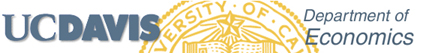Ph.D. Econometrics Sequence

*************

The following describes the Economics and Agricultural and Resource Economics Ph.D. Econometrics courses.
In addition some field courses such as ECN 230A taught by Marianne Bitler have a strong empirical econometrics component.

First year:

Fall:      239    Econometrics Foundations
Winter: 240A  Econometrics Methods I
Spring: 240B   Econometrics Methods II

Second year:

Fall:      240D or 240C   Cross-section Econometrics or Time Series Econometrics
Winter: 240D or 240C   Cross-section Econometrics or Time Series Econometrics
Spring: 240E    Topics in Time Series Econometrics
Winter: 240F    Topics in Cross-Section Econometrics
[Note: 240D is often taught the quarter before 240C.]

*************

Instructors for 2020-2021 are
239    Shu Shen (Fall)
240A  Aaron Smith (Winter)
240B  Dalia Ghanem (Spring)
****
240C  Bulat Gafarov (Fall)
240D  Takuya Ura  (Winter)
240E  Oscar Jorda (Spring)
240F  Colin Cameron  (Spring)

*************

The first-year sequence covers the basics and is required of all Economics and ARE Ph.D. students.

Much of the second-year sequence is also necessary for applied thesis research,
even though the courses are not formally  required.

Time-series is split into essentials (240C) and topics (240E).
Similarly cross-section is split into essentials (240D) and topics (240F).

For Economics students taking the econometrics field, the field is 240C, 240D and one of 240E/240F with grades of B+ or higher.

*************

Course Descriptions

239 Econometric Foundations
Lecture—3 hours; discussion—1 hour. Prerequisite: undergraduate statistics.
Statistical foundations for econometrics.

240A. Econometric Methods I
Lecture—3 hours; discussion—1 hour. Prerequisite: Economics 290 and a course in linear algebra or the equivalent.
Least squares, instrumental variables, and maximum likelihood estimation and inference for single equation linear regression model; finite sample and large sample theory; linear restrictions; heteroskedasticity; autocorrelation.

240B. Econometric Methods II
Lecture—3 hours; discussion—1 hour. Prerequisite: course 240A.
Maximum likelihood estimation and testing, nonlinear least squares, systems of equations, simultaneous equation models, introductory time series regression.

240C. Time Series Econometrics
Lecture—3 hours; discussion—1 hour. Prerequisite: course 240B. (Usually taught after 240D).
Probability theory; estimation, inference and forecasting of time series models; trends and non-standard asymptotic theory; vector time series methods and cointegration; time series models for higher order moments and transition data; state-space modeling and the Kalman filter.

240D. Cross Section Econometrics
Lecture – 3 hours; discussion – 1 hour. Prerequisite: course 240B. (Usually taught before 240C).
Estimation and inference for nonlinear regression models for cross-section data; models for discrete data and for limited dependent variables; models for panel data; additional topics such as bootstrap and semiparametric regression.

240E. Topics in Time Series Econometrics
Lecture—3 hours; discussion—1 hour. Prerequisite: courses 240A and 240B.
Modern econometric techniques for time series data. Expand on topics covered in Economics 240A, 240B, and 240C. Contents may vary from year to year.

240F. Topics in Cross Section Econometrics
Lecture—3 hours; discussion—1 hour. Prerequisite: courses 240A, 240B and 240D.
Modern econometric techniques for cross-section data. Expand on topics covered in Economics 240A, 240B, and 240D. Contents may vary from year to year.A. Colin Cameron / UC-Davis Economics /  http://cameron.econ.ucdavis.edu